Olympiad Test: Figure Puzzles

# Olympiad Test: Figure Puzzles

Test Description

## 20 Questions MCQ Test Science Olympiad Class 6 | Olympiad Test: Figure Puzzles

Olympiad Test: Figure Puzzles for Class 6 2022 is part of Science Olympiad Class 6 preparation. The Olympiad Test: Figure Puzzles questions and answers have been prepared according to the Class 6 exam syllabus.The Olympiad Test: Figure Puzzles MCQs are made for Class 6 2022 Exam. Find important definitions, questions, notes, meanings, examples, exercises, MCQs and online tests for Olympiad Test: Figure Puzzles below.
Solutions of Olympiad Test: Figure Puzzles questions in English are available as part of our Science Olympiad Class 6 for Class 6 & Olympiad Test: Figure Puzzles solutions in Hindi for Science Olympiad Class 6 course. Download more important topics, notes, lectures and mock test series for Class 6 Exam by signing up for free. Attempt Olympiad Test: Figure Puzzles | 20 questions in 40 minutes | Mock test for Class 6 preparation | Free important questions MCQ to study Science Olympiad Class 6 for Class 6 Exam | Download free PDF with solutions
 1 Crore+ students have signed up on EduRev. Have you?
Olympiad Test: Figure Puzzles - Question 1

### Directions: In this type of questions, a figure or a matrix is given in which some numbers are filled according to a rule. A place is left blank. You have to find out a character (a number or a letter) from the given possible answers which may be filled in the blank space. Q. Which one will replace the question mark?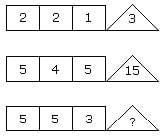Detailed Solution for Olympiad Test: Figure Puzzles - Question 1

From figure I  (2 × 2 – 1) = 3
and from figure II (5 × 4 – 5) = 15
From figure III (5 × 5 – 3) = 22

Olympiad Test: Figure Puzzles - Question 2

### Directions: In this type of questions, a figure or a matrix is given in which some numbers are filled according to a rule. A place is left blank. You have to find out a character (a number or a letter) from the given possible answers which may be filled in the blank space. Q.Which one will replace the question mark?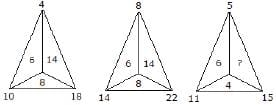Detailed Solution for Olympiad Test: Figure Puzzles - Question 2

For first triangle, 10 – 4 = 6
18 – 10 = 8
18 – 4 = 14
For second triangle, 14 – 8 = 6
22 – 14 = 8
22 – 8 = 14
For third triangle, 11 – 5 = 6
15 – 11 = 4
∴ ? = 15 – 5 = 10

Olympiad Test: Figure Puzzles - Question 3

### Directions: In this type of questions, a figure or a matrix is given in which some numbers are filled according to a rule. A place is left blank. You have to find out a character (a number or a letter) from the given possible answers which may be filled in the blank space. Q. Which one will replace the question mark?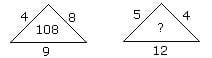Detailed Solution for Olympiad Test: Figure Puzzles - Question 3

From figure I  (4 + 8) × 9 = 108
∴ ? = (5 + 4) × 12 = 108

Olympiad Test: Figure Puzzles - Question 4

Directions: In this type of questions, a figure or a matrix is given in which some numbers are filled according to a rule. A place is left blank. You have to find out a character (a number or a letter) from the given possible answers which may be filled in the blank space.

Q. Which one will replace the question mark?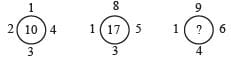Detailed Solution for Olympiad Test: Figure Puzzles - Question 4

1 + 2 + 3 + 4 = 10
and 1 + 3 + 5 + 8 = 17
Similarly, ? = 1 + 4 + 6 + 9 = 20

Olympiad Test: Figure Puzzles - Question 5

Directions: In this type of questions, a figure or a matrix is given in which some numbers are filled according to a rule. A place is left blank. You have to find out a character (a number or a letter) from the given possible answers which may be filled in the blank space.

Q. Which one will replace the question mark?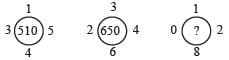Detailed Solution for Olympiad Test: Figure Puzzles - Question 5

From figure I  (1)2 + (5)2 + (4)2 + (3)2
=51 × 10 = 510
and from figure II (3)2 + (4)2 + (6)2 + (2)2
= 65 × 10 = 650
Similarly, from figure III (0)2 + (1)2 + (2)2 + (8)= 69 × 10 = 690

Olympiad Test: Figure Puzzles - Question 6

Directions: In this type of questions, a figure or a matrix is given in which some numbers are filled according to a rule. A place is left blank. You have to find out a character (a number or a letter) from the given possible answers which may be filled in the blank space.

Q. Which one will replace the question mark?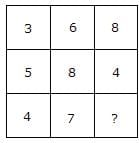Detailed Solution for Olympiad Test: Figure Puzzles - Question 6

From column I (5 + 3)/2 = 4
and From column II (6 + 8)/2 = 7
Therefore from column III ? = (8 + 4)/2 = 6

Olympiad Test: Figure Puzzles - Question 7

Directions: In this type of questions, a figure or a matrix is given in which some numbers are filled according to a rule. A place is left blank. You have to find out a character (a number or a letter) from the given possible answers which may be filled in the blank space.

Q. Which one will replace the question mark?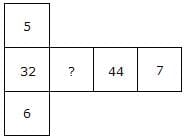Detailed Solution for Olympiad Test: Figure Puzzles - Question 7

Here,  (5 × 6) + 2 = 32
(7 × 6) + 2 = 44
∴  ? = (7 × 5) + 2 = 37

Olympiad Test: Figure Puzzles - Question 8

Directions: In this type of questions, a figure or a matrix is given in which some numbers are filled according to a rule. A place is left blank. You have to find out a character (a number or a letter) from the given possible answers which may be filled in the blank space.

Q. Which one will replace the question mark?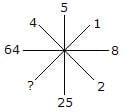Detailed Solution for Olympiad Test: Figure Puzzles - Question 8

Here, (2)2 = 4
(8)2 = 64
(5)2 = 25
∴ ? = (1)2 = 1

Olympiad Test: Figure Puzzles - Question 9

Directions: In this type of questions, a figure or a matrix is given in which some numbers are filled according to a rule. A place is left blank. You have to find out a character (a number or a letter) from the given possible answers which may be filled in the blank space.

Q. Which one will replace the question mark?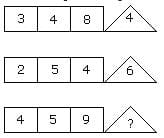Detailed Solution for Olympiad Test: Figure Puzzles - Question 9

From figure I (3 × 4 – 8) = 4
From figure II (2 × 5 – 4) = 6
and from figure III (4 × 5 – 9) = 11

Olympiad Test: Figure Puzzles - Question 10

Directions: In this type of questions, a figure or a matrix is given in which some numbers are filled according to a rule. A place is left blank. You have to find out a character (a number or a letter) from the given possible answers which may be filled in the blank space.

Q. Which one will replace the question mark?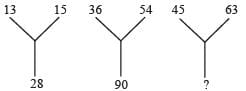Detailed Solution for Olympiad Test: Figure Puzzles - Question 10

From figure I 13 + 15 = 28
From figure II 36 + 54 = 90
Therefore, from figure III 45 + 63 = 108

Olympiad Test: Figure Puzzles - Question 11

Directions: In this type of questions, a figure or a matrix is given in which some numbers are filled according to a rule. A place is left blank. You have to find out a character (a number or a letter) from the given possible answers which may be filled in the blank space.

Q. Which one will replace the question mark?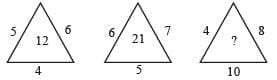Detailed Solution for Olympiad Test: Figure Puzzles - Question 11

From figure I (5 × 6 × 4)/10 = 12 and from figure II (6 × 7 × 5)/10 = 21 Therefore, from figure III ? = (4 × 8 × 10)/10 = 32

Olympiad Test: Figure Puzzles - Question 12

Directions: In this type of questions, a figure or a matrix is given in which some numbers are filled according to a rule. A place is left blank. You have to find out a character (a number or a letter) from the given possible answers which may be filled in the blank space.

Q. Which one will replace the question mark?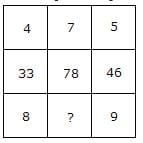Detailed Solution for Olympiad Test: Figure Puzzles - Question 12

From column I (4 × 8) + 1 = 33
From column II (5 × 9) + 1 = 46
Similarly, from column III (7 × ?) + 1  = 78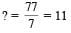Olympiad Test: Figure Puzzles - Question 13

Directions: In this type of questions, a figure or a matrix is given in which some numbers are filled according to a rule. A place is left blank. You have to find out a character (a number or a letter) from the given possible answers which may be filled in the blank space.

Q. Which one will replace the question mark?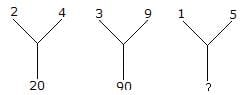Detailed Solution for Olympiad Test: Figure Puzzles - Question 13

(2)2 + (4)2 = 20
(3)2 + (9)2 = 90
Therefore, ? = (1)2 + (5)2 = 26

Olympiad Test: Figure Puzzles - Question 14

Directions: In this type of questions, a figure or a matrix is given in which some numbers are filled according to a rule. A place is left blank. You have to find out a character (a number or a letter) from the given possible answers which may be filled in the blank space.

Q. Which one will replace the question mark?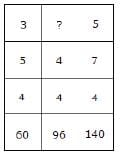Detailed Solution for Olympiad Test: Figure Puzzles - Question 14

3 × 5 × 4 = 60
and 5 × 7 × 4 = 140
Therefore, 4 × 4 × ? = 96
⇒ ? = (96/16) = 6

Olympiad Test: Figure Puzzles - Question 15

Directions: In this type of questions, a figure or a matrix is given in which some numbers are filled according to a rule. A place is left blank. You have to find out a character (a number or a letter) from the given possible answers which may be filled in the blank space.

Q. Which one will replace the question mark?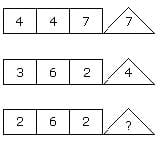Detailed Solution for Olympiad Test: Figure Puzzles - Question 15

(4 × 7) ÷ 4 = 7
and (6 × 2) ÷ 3 = 4
Therefore, (6 × 2) ÷ 2 = 6

Olympiad Test: Figure Puzzles - Question 16

Directions: In this type of questions, a figure or a matrix is given in which some numbers are filled according to a rule. A place is left blank. You have to find out a character (a number or a letter) from the given possible answers which may be filled in the blank space.

Q. Which one will replace the question mark?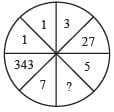Detailed Solution for Olympiad Test: Figure Puzzles - Question 16

All numbers are cubes,
(7)3 = 343
(1)3 = 1
(3)3 = 27
Similarly, ? = (5)3 = 125

Olympiad Test: Figure Puzzles - Question 17

Directions: In this type of questions, a figure or a matrix is given in which some numbers are filled according to a rule. A place is left blank. You have to find out a character (a number or a letter) from the given possible answers which may be filled in the blank space.

Q. Which one will replace the question mark?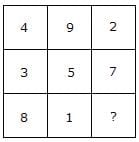Detailed Solution for Olympiad Test: Figure Puzzles - Question 17

Here,
(4 + 9 + 2) = (3 + 5 + 7) = (8 + 1 + ?)
⇒ ? = 15 – 9 = 6
Total in each case = 15

Olympiad Test: Figure Puzzles - Question 18

Directions: In this type of questions, a figure or a matrix is given in which some numbers are filled according to a rule. A place is left blank. You have to find out a character (a number or a letter) from the given possible answers which may be filled in the blank space.

Q. Which one will replace the question mark?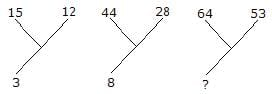Detailed Solution for Olympiad Test: Figure Puzzles - Question 18

(15 + 12)/9 = 3
and (44 + 28)/9 = 8 Therefore, ? = (64 + 53)/9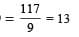Olympiad Test: Figure Puzzles - Question 19

Directions: In this type of questions, a figure or a matrix is given in which some numbers are filled according to a rule. A place is left blank. You have to find out a character (a number or a letter) from the given possible answers which may be filled in the blank space.

Q. Which one will replace the question mark?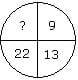Detailed Solution for Olympiad Test: Figure Puzzles - Question 19

Here,
9 + (2)2 = 13
and 13 + (3)2 = 22
and ? = 22 + (4)2 = 38

Olympiad Test: Figure Puzzles - Question 20

Directions: In this type of questions, a figure or a matrix is given in which some numbers are filled according to a rule. A place is left blank. You have to find out a character (a number or a letter) from the given possible answers which may be filled in the blank space.

Q. Which one will replace the question mark?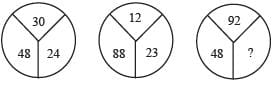Detailed Solution for Olympiad Test: Figure Puzzles - Question 20

(30 – 24) × 8 = 48
and (23 – 12) × 8 = 88
Therefore, (92 – ?) × 8 = 48
⇒ 92 – ? = 6
⇒ ? = 92 –6 = 86

## Science Olympiad Class 6

16 videos|42 tests
 Use Code STAYHOME200 and get INR 200 additional OFF Use Coupon Code
Information about Olympiad Test: Figure Puzzles Page
In this test you can find the Exam questions for Olympiad Test: Figure Puzzles solved & explained in the simplest way possible. Besides giving Questions and answers for Olympiad Test: Figure Puzzles, EduRev gives you an ample number of Online tests for practice

## Science Olympiad Class 6

16 videos|42 tests